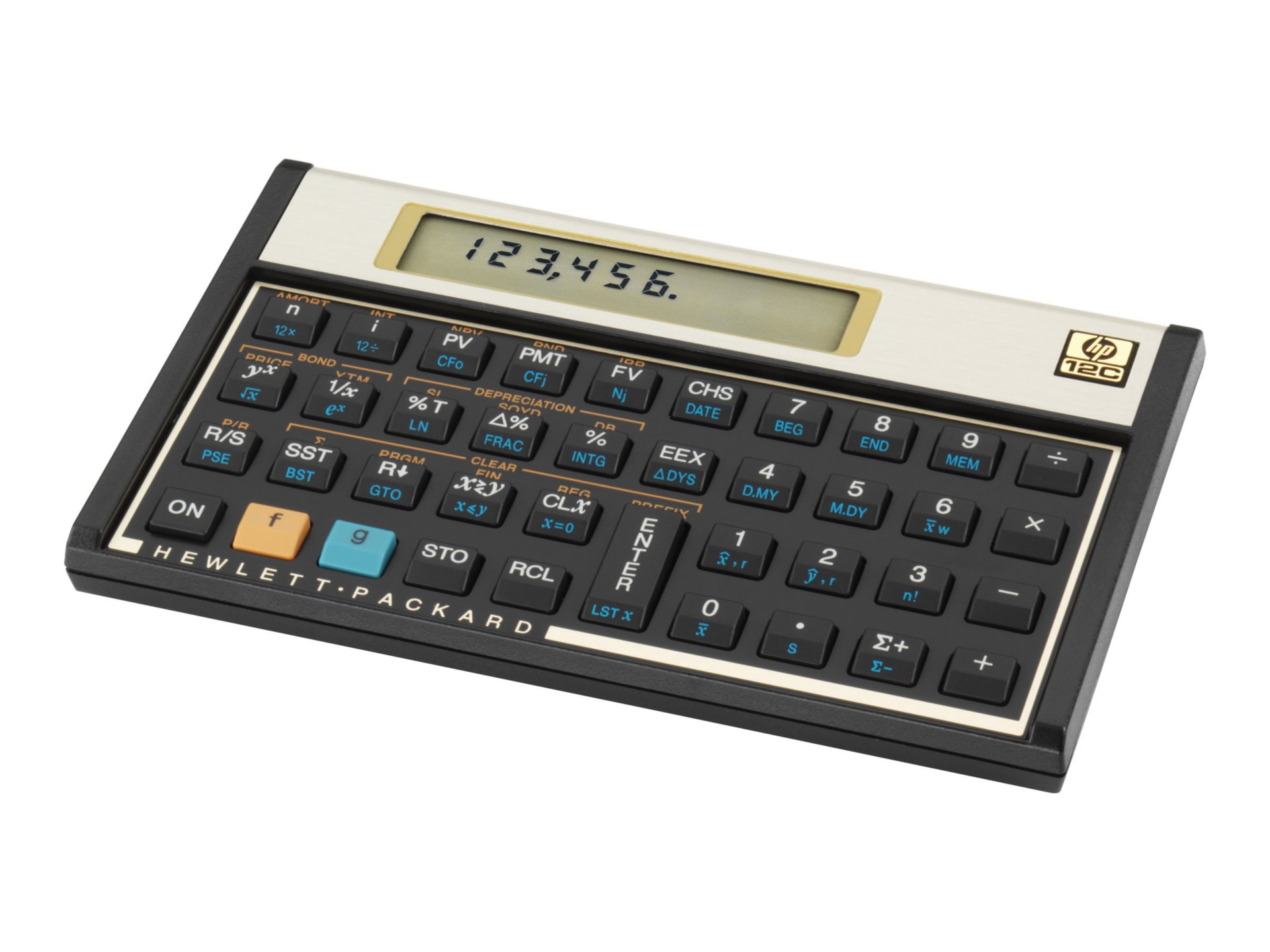# HP 12C Financial Calculator

Mfg.Part: 12C#ABA | CDW Part: 154342 | UNSPSC: 44101800
Availability: In Stock
Warranties
Advertised Price
Better TogetherTotal Price:

### Product Details

The 12c is the industry staple in financial calculators. Millions of users the world over rely on this time-tested performer. Millions of users know that the 12c has the power to ensure that its more than 120 built-in functions are available when you need them. This calculator is the smart choice, the industry’s choice.

Features
Efficient RPN data entry

Over 120 built-in functions

Calculates NPV, IRR, TVM, cash flows, bonds, amortization, and depreciation.

Calculates bond price and yield to maturity

Calculates linear regression, standard deviation, mean, and weighted mean

Cumulative statically analysis

Allowed on the CFP, CFA, and Capital exams

Formulas:
TVM (loans, savings and leasing)
Amoritization
Bond price and yield to maturity
Register based cash flow analysis NPV, IRR
Memory for 20 cash flows
SL, DB, SOYD depreciation methods
% change, % of total
Keystroke programming
Memory capacity: 99 steps
Entry system logic
Cumulative statistical analysis
Std. deviation, mean, weighed mean
Linear regression
Forecasting, correlation coefficient
Date arithmetic

Specifications:
Dimensions: 5"x3.1"x0.6"

What's in the Box?
Owner's Manual
Batteries
Carrying Case